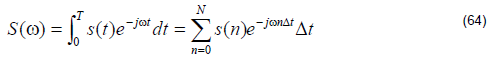As a time domain simulation method; FDTD can get all the relevant spectral response
with a single simulation. To get the spectral response, you need to use the DFT, FFT,
and Analysis. Discretized Fourier Transform gets a single wavelength response from
a time series.where s(n) are the time domain response, N is the number of time steps, and ω is
the angle frequency.

When DFT is running in the simulator, it will get the frequency domain response only
for the center wavelength, while DFT for the observation point, area, and line gives
the spectral response for a series of wavelengths which are identified by the user.

Fast Fourier Transform uses the traditional fast Fourier transform scheme to get a
spectral response from the zero frequency to the cutoff frequency 1 ⁄ (2Dt); and the
frequency domain sampling step is 1 ⁄ (2NDt).

In general, the sampling frequency step for FFT is comparable to the wavelength due
to the fact that the FDTD required time step is very small. Therefore, the FFT results
may have a larger error than that of the DFT results, but the FFT method is much
faster than DFT.